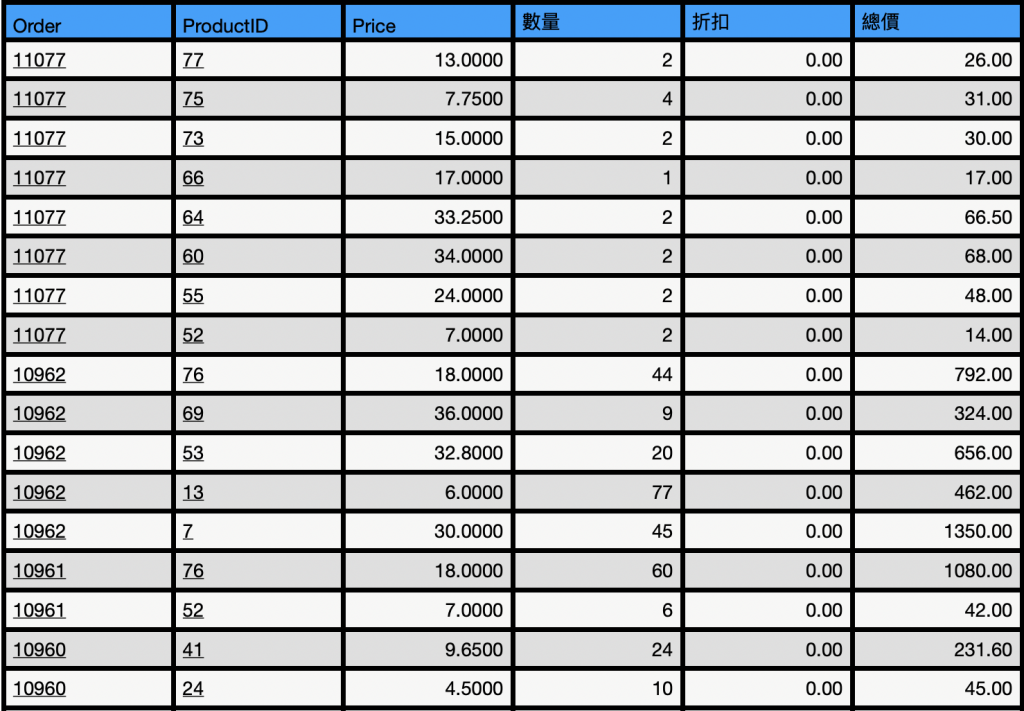0

[MySQL]請問如何依照相同Order編號 , 使總價相加, 並且只輸出單一Order編號4 個回答

0

SELECT DENSE_RANK() OVER(ORDER BY SUM([總價]) DESC) AS NO, [Order] AS Order, SUM([總價]) AS Total_Price
FROM [Table_Name]
group by [Order]

3
select Order as order_id, SUM('總價') as amount from table_name group by order_id
0

MSSQL

select [Order],SUM([總價]) Total
from Ordertable
group by [Order]
0

DEMO

select order_num , SUM(total_price)
from sales
group by order_num;